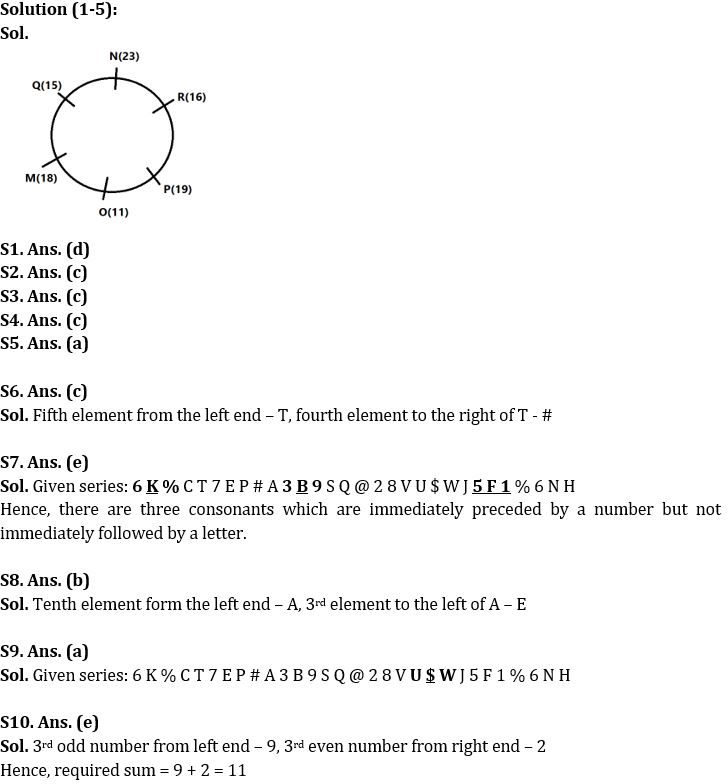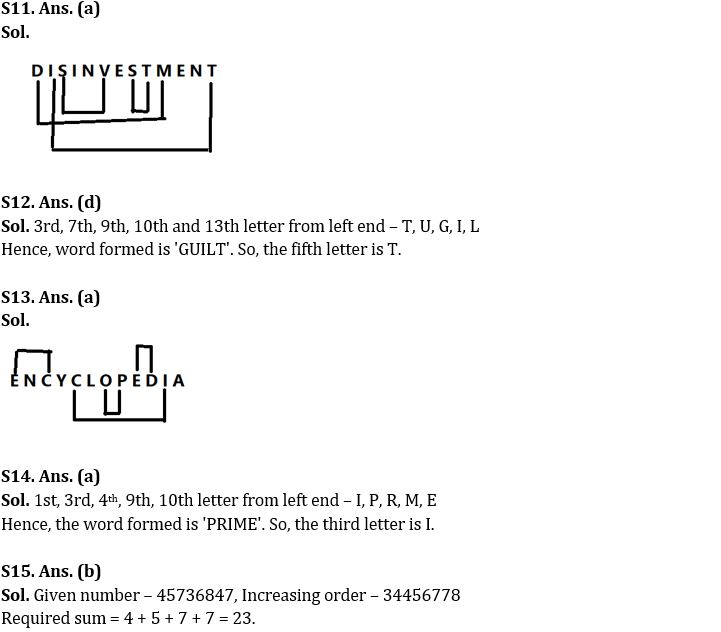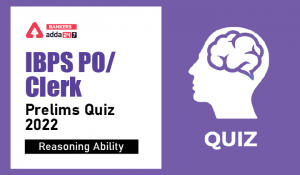Latest Banking jobs   »

# Reasoning Ability Quiz For IBPS RRB PO Clerk Prelims 2022- 28th July

Directions (1-5): Answer the questions based on the information given below:
Six persons M, N, O, P, Q and R sit around a circular table facing towards the center. Each of them is certain years old. Distance between adjacent persons is equal. Sum of ages of the persons sitting opposite to each other is 34 years. None of them is lesser than 11 years old and greater than 23 years old.
P sits opposite to 15 years old person. One person sits between P and the one who is 4 years elder than P. R sits 2nd to the right of the youngest person. M is two years elder than R whose age is an even number. Q sits immediate right of N.

Q1. Who sits immediate left of M?
(a) R
(b) P
(c) O
(d) Q
(e) None of these

Q2. Person who is 16 years old sits 2nd to the right of which of following person?
(a) R
(b) P
(c) O
(d) Q
(e) None of these

Q3. Who sits opposite to N?
(a) R
(b) P
(c) O
(d) M
(e) None of these

Q4. What is the sum of ages of R and O?
(a) 29
(b) 28
(c) 27
(d) 31
(e) None of these

Q5. Who sits immediate right of the eldest person?
(a) Q
(b) P
(c) M
(d) R
(e) None of these

Directions (6-10): Study the given arrangement of numbers, symbols and alphabets and answer the questions based on it.

6 K % C T 7 E P # A 3 B 9 S Q @ 2 8 V U \$ W J 5 F 1 % 6 N H

Q6. Which of the following is fourth element to the right of fifth element from the left end of the given arrangement?
(a) C
(b) F
(c) #
(d) S
(e) None of the above

Q7. How many such consonants are immediately preceded by a number but not immediately followed by a letter?
(a) One
(b) Four
(c) Two
(d) None
(e) None of the above

Q8. Which of the following element is 3rd to the left of 10th element from left end in the given series?
(a) P
(b) E
(c) J
(d) N
(e) None of the above

Q9. How many symbols are immediately followed by a letter but not immediately preceded by a consonant?
(a) One
(b) Four
(c) Two
(d) Three
(e) None of the above

Q10. In the given arrangement what is the sum of 3rd odd number from left end and 3rd even number from right end?
(a) 12
(b) 14
(c) 18
(d) 10
(e) 11

Q11. How many pairs of letters are there in the word ‘DISINVESTMENT‘ which has as many letters between them as in English alphabetical series (both backward and forward direction)?
(a) Four
(b) Five
(c) Six
(d) Seven
(e) None of the above

Q12. What is the fifth letter of the meaningful word formed using 3rd, 7th, 9th, 10th & 13th letter from left end of the word ‘METALLURGICAL‘?
(a) L
(b) I
(c) G
(d) T
(e) None of the above

Q13. How many pairs of letters are there in the word ‘ENCYCLOPEDIA‘ which has as many letters between them as in English alphabetical series (both backward and forward direction)?
(a) Four
(b) Five
(c) Six
(d) Three
(e) More than six

Q14. What is the third letter of the meaningful word formed using 1st, 3rd, 4th, 9th and 10th letter from left end of the word ‘IMPRISONMENT‘?
(a) I
(b) E
(c) R
(d) M
(e) None of the above

Q15. If digits of the number “45736847” are arranged in increasing order from left to right, then what will be the sum of 2nd, 4th, 6th, and 7th digits (from the left end) of the number so formed?
(a) 32
(b) 23
(c) 28
(d) 24
(e) None of the above

Solutions#### Congratulations!Incorrect details? Fill the form again here

•Quantitative Aptitude Quiz For IBPS RRB ...
•Reasoning Ability Quiz For IBPS RRB PO C...
•Quantitative Aptitude Quiz For IBPS RRB ...
•Reasoning Ability Quiz For IBPS RRB PO C...
•Reasoning Ability Quiz For IBPS Clerk/PO...
•Quantitative Aptitude Quiz For IBPS RRB ...